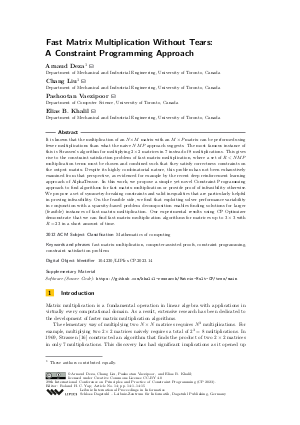Document# Fast Matrix Multiplication Without Tears: A Constraint Programming Approach

### Authors Arnaud Deza, Chang Liu, Pashootan Vaezipoor, Elias B. Khalil## File

LIPIcs.CP.2023.14.pdf
• Filesize: 0.69 MB
• 15 pages

## Cite As

Arnaud Deza, Chang Liu, Pashootan Vaezipoor, and Elias B. Khalil. Fast Matrix Multiplication Without Tears: A Constraint Programming Approach. In 29th International Conference on Principles and Practice of Constraint Programming (CP 2023). Leibniz International Proceedings in Informatics (LIPIcs), Volume 280, pp. 14:1-14:15, Schloss Dagstuhl - Leibniz-Zentrum für Informatik (2023)
https://doi.org/10.4230/LIPIcs.CP.2023.14

## Abstract

It is known that the multiplication of an N × M matrix with an M × P matrix can be performed using fewer multiplications than what the naive NMP approach suggests. The most famous instance of this is Strassen’s algorithm for multiplying 2× 2 matrices in 7 instead of 8 multiplications. This gives rise to the constraint satisfaction problem of fast matrix multiplication, where a set of R < NMP multiplication terms must be chosen and combined such that they satisfy correctness constraints on the output matrix. Despite its highly combinatorial nature, this problem has not been exhaustively examined from that perspective, as evidenced for example by the recent deep reinforcement learning approach of AlphaTensor. In this work, we propose a simple yet novel Constraint Programming approach to find algorithms for fast matrix multiplication or provide proof of infeasibility otherwise. We propose a set of symmetry-breaking constraints and valid inequalities that are particularly helpful in proving infeasibility. On the feasible side, we find that exploiting solver performance variability in conjunction with a sparsity-based problem decomposition enables finding solutions for larger (feasible) instances of fast matrix multiplication. Our experimental results using CP Optimizer demonstrate that we can find fast matrix multiplication algorithms for matrices up to 3× 3 with R = 23 in a short amount of time.

## Subject Classification

##### ACM Subject Classification
• Mathematics of computing
##### Keywords
• fast matrix multiplication
• computer-assisted proofs
• constraint programming
• constraint satisfaction problem

## Metrics

• Access Statistics
• Total Accesses (updated on a weekly basis)
0

## References

1. Austin R. Benson and Grey Ballard. A framework for practical parallel fast matrix multiplication. ACM SIGPLAN Notices, 50(8):42-53, January 2015. URL: https://doi.org/10.1145/2858788.2688513.
2. Markus Blaser. On the complexity of the multiplication of matrices of small formats. Journal of Complexity, 19(1):43-60, 2003.3. Markus Bläser. Fast Matrix Multiplication. Number 5 in Graduate Surveys. Theory of Computing Library, 2013. URL: https://doi.org/10.4086/toc.gs.2013.005.
4. Roger W Brockett and David Dobkin. On the optimal evaluation of a set of bilinear forms. In Proceedings of the fifth annual ACM symposium on Theory of computing, pages 88-95, 1973.5. Nicolas T Courtois, Gregory V Bard, and Daniel Hulme. A new general-purpose method to multiply 3x3 matrices using only 23 multiplications, 2011.6. Hans F de Groote. On varieties of optimal algorithms for the computation of bilinear mappings ii. optimal algorithms for 2× 2-matrix multiplication. Theoretical Computer Science, 7(2):127-148, 1978.7. Alhussein Fawzi, Matej Balog, Aja Huang, Thomas Hubert, Bernardino Romera-Paredes, Mohammadamin Barekatain, Alexander Novikov, Francisco J R Ruiz, Julian Schrittwieser, and Grzegorz Swirszcz. Discovering faster matrix multiplication algorithms with reinforcement learning. Nature, 610(7930):47-53, 2022.8. Matteo Fischetti and Michele Monaci. Exploiting erraticism in search. Operations Research, 62(1):114-122, 2014.9. Ambros Gleixner, Gregor Hendel, Gerald Gamrath, Tobias Achterberg, Michael Bastubbe, Timo Berthold, Philipp Christophel, Kati Jarck, Thorsten Koch, Jeff Linderoth, et al. Miplib 2017: data-driven compilation of the 6th mixed-integer programming library. Mathematical Programming Computation, 13(3):443-490, 2021.10. Marijn JH Heule, Manuel Kauers, and Martina Seidl. Local search for fast matrix multiplication. In Theory and Applications of Satisfiability Testing-SAT 2019: 22nd International Conference, SAT 2019, Lisbon, Portugal, July 9-12, 2019, Proceedings 22, pages 155-163. Springer, 2019.11. Marijn JH Heule, Manuel Kauers, and Martina and Seidl. New ways to multiply 3 × 3-matrices. Journal of Symbolic Computation, 104:899-916, 2021.12. Andrea Lodi and Andrea Tramontani. Performance variability in mixed-integer programming. In Theory driven by influential applications, pages 1-12. INFORMS, 2013.13. Alexey V. Smirnov. The bilinear complexity and practical algorithms for matrix multiplication. Computational Mathematics and Mathematical Physics, 53:1781-1795, 2013.14. Laurent Sorber and Marc Van Barel. A mixed-integer linear program formulation for fast matrix multiplication, 2017.15. David Speck, Paul Höft, Daniel Gnad, and Jendrik Seipp. Finding matrix multiplication algorithms with classical planning. In Sven Koenig, Roni Stern, and Mauro Vallati, editors, Proceedings of the Thirty-Third International Conference on Automated Planning and Scheduling (ICAPS 2023). AAAI Press, 2023.16. Volker Strassen. Gaussian elimination is not optimal. Numerische mathematik, 13(4):354-356, 1969.17. Shmuel Winograd. On the number of multiplications necessary to compute certain functions. Communications on Pure and Applied Mathematics, 23(2):165-179, 1970.X

Feedback for Dagstuhl Publishing